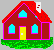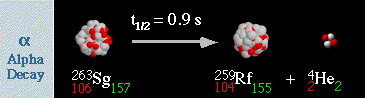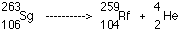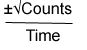# EXPERIMENT #2: ALPHA PLEASE LEAVE HOME

## Introduction

An alphaparticle is a nucleus of a helium-4 atom. It has two protons and two neutrons with an atomic mass of 4. The new nucleus that results from alpha decay will have a mass and charge different from those of the parent nucleus. A nucleus which undergoes alpha decay transforms into a new element. This process is called transmutation.

EXAMPLEThe atomic number changes from 106 to 104. Measurements show that the sum of the masses of the daughter nucleus and the alpha particle is less than the mass of the parent isotope. Recalling Einstein's formula E=mc2, this loss of mass is converted into energy. This form of energy is a positively charged particle moving at high speed. It is easily stopped by paper or your hand.
In this experiment the distances are 0.5 cm, 1.0 cm, 1.5 cm, and 2.0 cm. Data point one equals 0.5 cm. Thus data point two equals 1.0 cm and so on.

## Objective

The purpose of this experiment is to find the range of alpha particles and determine if the inverse square law applies.

## Materials

• Geiger counter
• rail tracker
• Po-210 (alpha source)
• stop watch/beeper
• counting paper or hand counter
• graph paper

## Procedure

1. Place alpha radiation source into hole on wooden block of rail tracker.
2. Set digital Geiger counter to one minute intervals and turn power on. Allow the instrument to warm up for a few minutes.
3. Record background activity.
4. Place the instrument on the slider, 0.5 cm from the source.
5. Record counts per minute (cpm) in Table 2.1 for each trial and calculate the average.
6. Move Geiger counter and slider to 1.0 cm from source. Repeat step 5.
7. Move Geiger counter to 1.5 cm and repeat step 5.
8. Move Geiger counter to 2.0 cm and again repeat step 5.
9. Calculate the uncertainty *. Record the calculation in column 8 of Table 2.1. The number following the plus or minus will indicate the error of the measurement.

 *Formula for the uncertainty of the number of counts Uncertainty (counts / time) =## Data

Distance (cm) Data Points r2 Trial 1 Trial 2 Trial 3 Average (cpm) Uncertainty (cpm) (1/r2) average count of first data point
0.5 1 1
1.0 2 4
1.5 3 9
2.0 4 16

1. Graph the activity readings (cpm) vs. distance.
2. Graph the activity readings (cpm) vs. 1/r2.

## Questions

1. At what distance did the alpha radiation count equal that of the background count?
2. What is the charge of the alpha particle? How do you know this?
3. List several reasons why the alpha particle does not travel more than several centimeters.

## Going Further

1. What is the mass of an alpha particle compared to an electron?
2. Using "Chart of Nuclides," what distinguishes the daughter isotopes and the particles emitted from each other?

This page was created by the Nuclear Science Division of the Lawrence Berkeley National Laboratory.